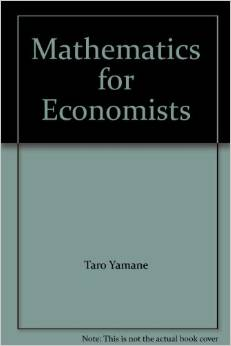IT IS TARO YAMANE NOT YARO YAMANE (ALSO LEARN HOW TO CALCULATE SAMPLE SIZE USING THE FORMULA)

December 29, 2014 By Chidi Rafael

Ever used a popular formula to determine your sample size? Ya! thats the dude am talking about.-The great mathematician and statistician combined. If you have done a research project or currently conducting one, you will definitely come across this great statistician called Taro Yamane.

If you are also a good observer, you will observe that his name is often 'abused' by numerous scholars. I mean i have read numerous research journals and articles where he is being referenced as 'Yaro Yamane'. Now let me shock you a bit. Its actually Taro not Yaro. So if you referenced him as Yaro Yamane in your previous research, then you referenced the wrong man. If you are currently conducting a research study please take note. Below are cover pages of some of his books to fully convince you.

How to Calculate Sample Size Using Taro Yamane's Formula:

For the purpose of this tutorial, let us use a simple survey-an organization in Uyo, Nigeria with a population of 82 Staff(Both managerial, and Junior staff).

3.4   Sample Size and Sampling Techniques
As a result of the inability of the researcher to effectively study the whole staff strength (population) of the organisation, a representative number was chosen as the sample size population. Sixty eight (68) staff was used as the sample size. The sample size was calculated using the Taro Yamane scientific formula which is given as:

n =             N
1 + N (e) 2

Where:
N is the Population (82 was the Population for the Study)
1  is the constant
e  is the degree of error expected
n  is the sample size?

n =                                     821 + 82 (0.05)2

821 + 82 (0.0025)

821 + 0.205

821 .205

n = 68

YOU CAN ALSO USE OUR SAMPLE SIZE CALCULATOR BELOW TO CALCULATE YOUR SAMPLE SIZE IN SECONDS!

Click To Calculate Sample Size ?